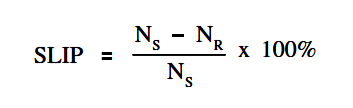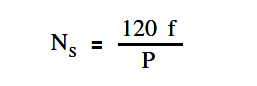# AC Induction Motor Slip

It is virtually impossible for the rotor of an AC induction motor to turn at the same speed as that of the rotating magnetic field. If the speed of the rotor were the same as that of the stator, no relative motion between them would exist, and there would be no induced EMF in the rotor. (Recall from earlier article that relative motion between a conductor and a magnetic field is needed to induce a current.) Without this induced EMF, there would be no interaction of fields to produce motion. The rotor must, therefore, rotate at some speed less than that of the stator if relative motion is to exist between the two.

The percentage difference between the speed of the rotor and the speed of the rotating magnetic field is called slip. The smaller the percentage, the closer the rotor speed is to the rotating magnetic field speed. Percent slip can be found by using below Equation.where

NS = synchronous speed (rpm)
NR = rotor speed (rpm)

The speed of the rotating magnetic field or synchronous speed of a motor can be found by using below Equation.where

NS = speed of rotating field (rpm)
f = frequency of rotor current (Hz)
P = total number of poles

Example:

A two pole, 60 Hz AC induction motor has a full load speed of 3554 rpm. What is the percent slip at full load?

Solution:

Synchronous speed:NS = (120 x 60 )/2

NS = 3600 rpm

Slip:Slip = { (3600 – 3554) / 3600 } 100

Slip = 1.3 %